# Digital Electronics - Combinational Logic Analysis

### Exercise :: Combinational Logic Analysis - General Questions

6.

Before an SOP implementation, the expression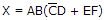would require a total of how many gates?

 A. 1 B. 2 C. 4 D. 5

Answer: Option D

Explanation:

No answer description available for this question. Let us discuss.

7.

The following waveform pattern is for a(n) ________.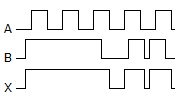A. 2-input AND gate B. 2-input OR gate C. Exclusive-OR gate D. None of the above

Answer: Option B

Explanation:

No answer description available for this question. Let us discuss.

8.

Implementing the expression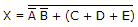with NOR logic, we get: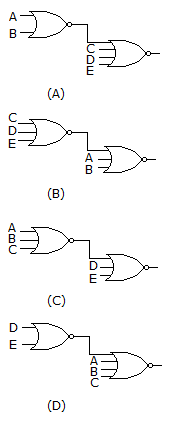A. (A) B. (B) C. (C) D. (D)

Answer: Option A

Explanation:

No answer description available for this question. Let us discuss.

9.

A 4-variable AND-OR-Invert circuit produces a 0 at its Y output. Which combination of inputs is correct?

 A.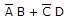B.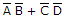C.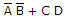D. none of the above

Answer: Option C

Explanation:

No answer description available for this question. Let us discuss.

10.

The following waveform pattern is for a(n) ________.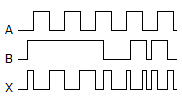A. 2-input AND gate B. 2-input OR gate C. Exclusive-OR gate D. None of the above

Answer: Option C

Explanation:

No answer description available for this question. Let us discuss.

#### Current Affairs 2021

Interview Questions and Answers